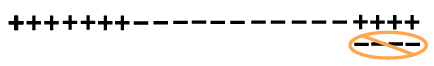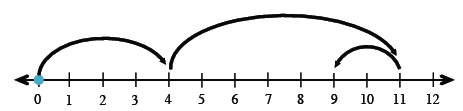Home > ACC6 > Chapter cc24 > Lesson cc24.1.1 > Problem4-6

4-6.

Simplify the expressions below. Homework Help ✎

1. $8 - 13 - ( - 4 )$$−1$

2. $4 - ( - 7 ) + ( - 2 )$3. $- 7 + 3 - 20$

If you start at $0$, move to left $7$, right $3$, and left $20$, where do you end up?